# Original WhisperFin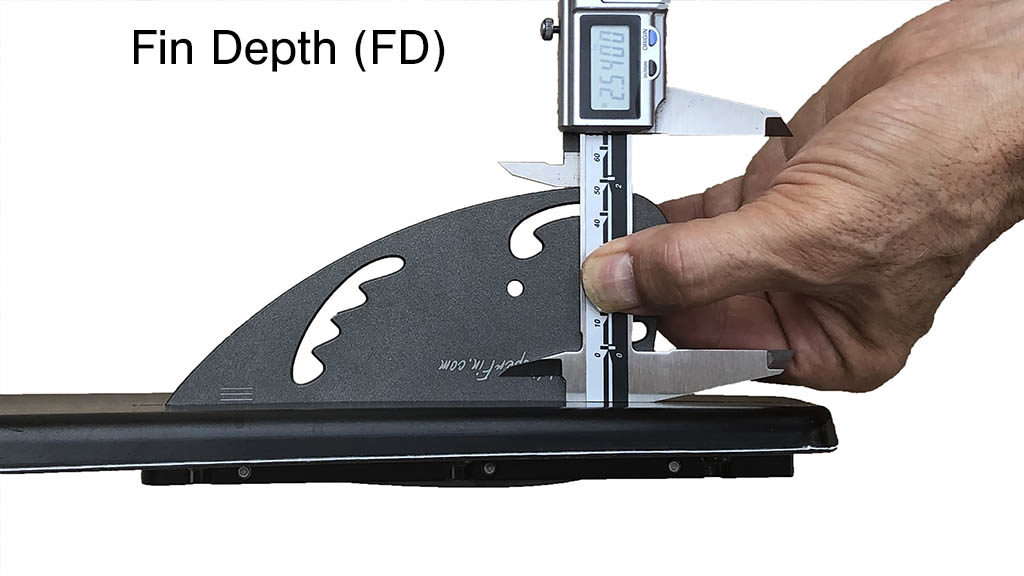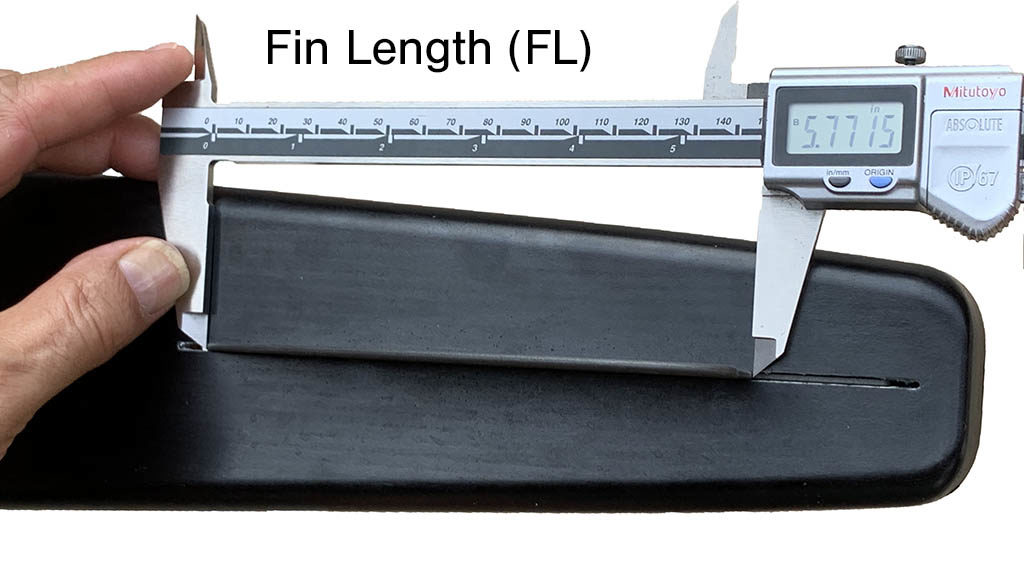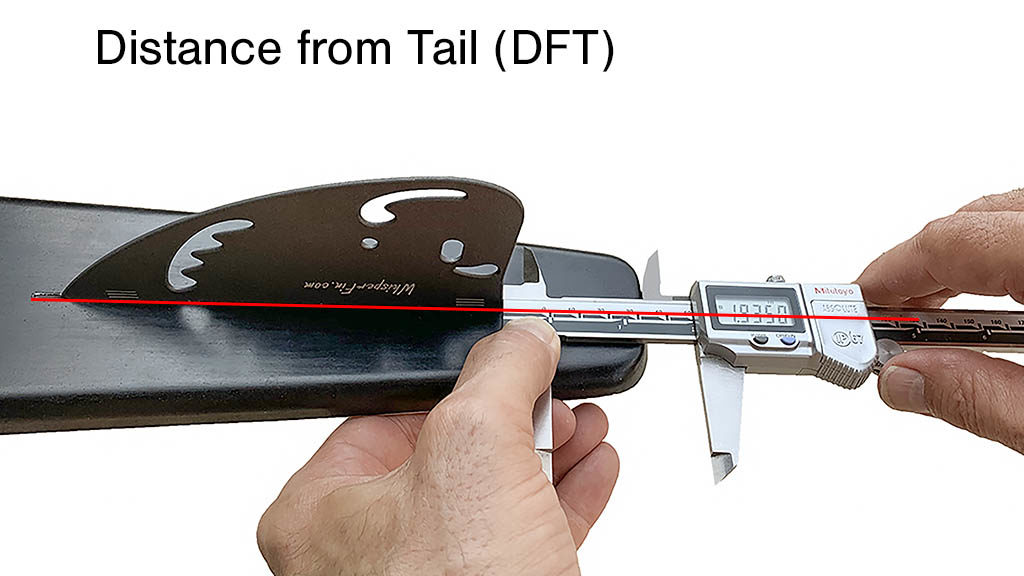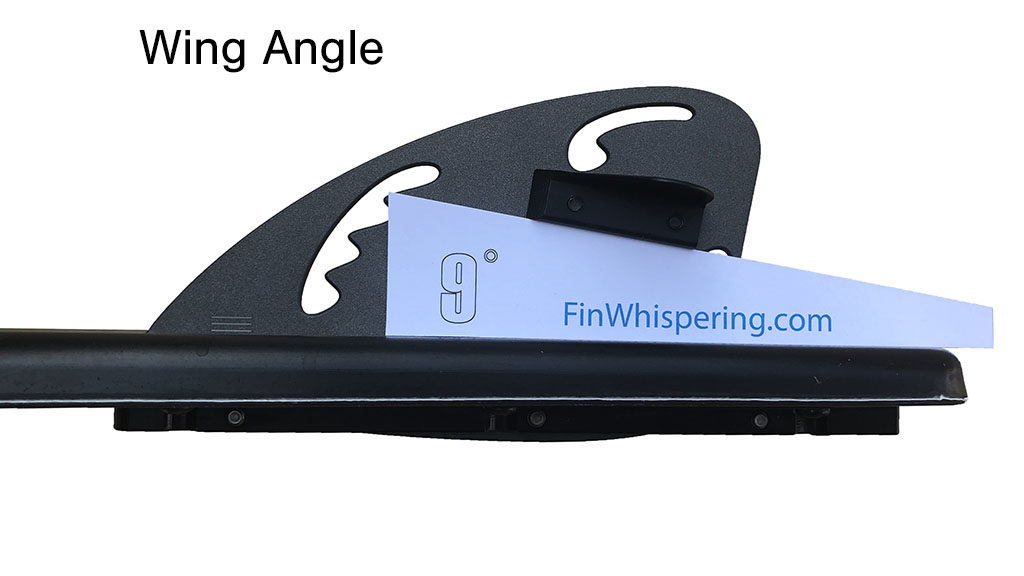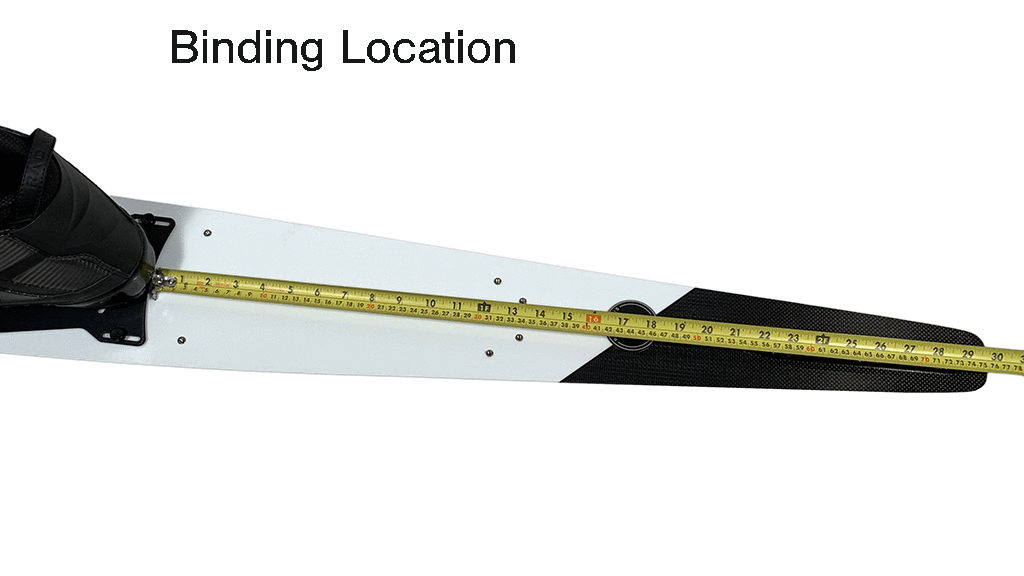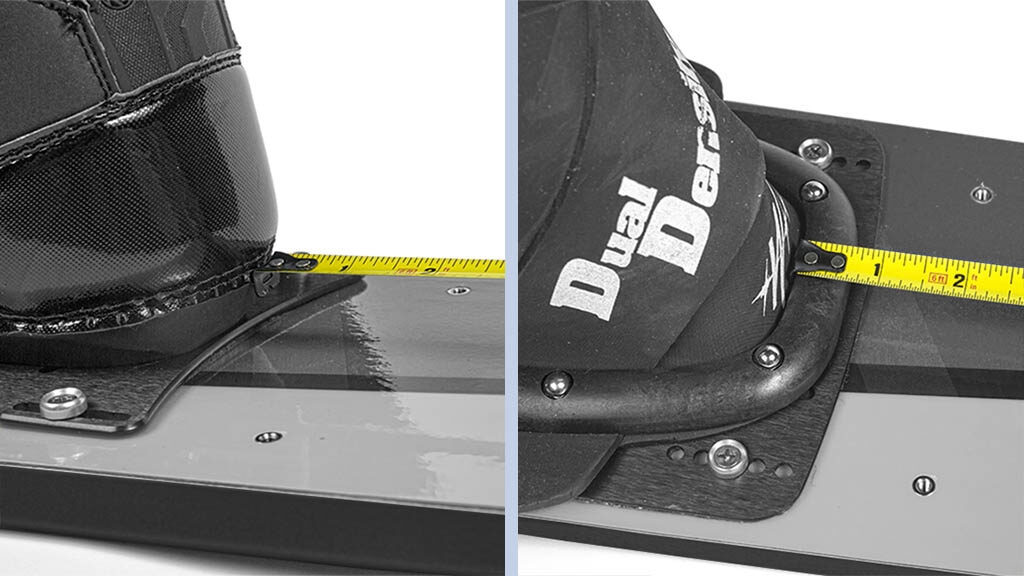The measurements listed in this database were taken using these industry standard techniques

• Move your bindings ½” for every inch of ski length difference (e.g. If the listed ski 1″ larger than yours, move your bindings forward ½”).
• Change DFT .005″ for every inch of ski length difference (e.g. If the listed ski 1″ larger than yours, increase DFT by .005″).

## Original Fin Starting Setups by Ski Brand

##### Connelly Installation Note:

With Connelly’s unique new fin block, the adjustment set screws contact the top sheet of the ski before you can achieve the desired FD and FL with the WhisperFin. This is not a problem. You can either adjust the fin by hand without the use of the set screws, Dremel out a round hole through the top sheet for the set screws to pass through (do not use a standard drill bit as it may snag and crack the top sheet). Or you can simply force the set screws through the top sheet into the foam core (this works fine and does no structural or cosmetic damage).* Notice the significant difference between the 66″ and 67″ DFT measurements. The 67″ setup was for a 35-off skier who was struggling with too much tip-bite. This short DFT strategy will work to reduce tip-grab for any sized ski. And note that it’s not the short DFT that reduces tip-bite, it’s the short leading edge location (LE).

## GT-R

Original Fin

Nimble
Bf = 28.625
Wing = 8°
FD = 2.540
FL = 5.770
DFT = 1.950
LE = 7.720

Supportive
Bf = 29.125
Wing = 8°
FD = 2.530
FL = 5.760
DFT = 1.905
LE = 7.665

#### 67″

Anti Tip-grab
Bf = 29.625
Wing = 7°
FD = 2.530
FL = 5.760
DFT = 1.805*
LE = 7.565**

*The front (FL) set screw must be removed to achieve this DFT
**This setup can be made nimbler by increasing DFT by up to .080″  or until the tip starts grabbing

Original Fin

Supportive
Bf = 29.375
Wing = 7°
FD = 2.510
FL = 5.750
DFT = 1.880
LE = 7.630

Original Fin

Nimble
Bf = 29.375
Wing = 7°
FD = 2.530
FL = 5.762
DFT = 1.950
LE = 7.712

Supportive
Bf = 29.5
Wing = 7°
FD = 2.540
FL = 5.770
DFT = 1.920
LE = 7.690

Original Fin

Bf = 28.625
Wing = 7°
FD = 2.485
FL = 5.730
DFT = 1.945
LE = 7.675

Bf = 29.25
Wing = 7°
FD = 2.520
FL = 5.755
DFT = 1.920
LE = 7.675

Bf = 29.75
Wing = 7°
FD = 2.530
FL = 5.762
DFT = 1.920
LE = 7.682

Bf = 30.125
Wing = 8°
FD = 2.530
FL = 5.760
DFT = 1.925
LE = 7.685

Bf = 30.625
Wing = 7°
FD = 2.540
FL = 5.770
DFT = 1.920
LE = 7.690

Original Fin

Bf = 29.65
Wing = 7°
FD = 2.525
FL = 5.760
DFT = 1.945
LE = 7.705

Bf = 30.15
Wing = 7°
FD = 2.530
FL = 5.760
DFT = 1.955
LE = 7.715

Bf = 30.65
Wing = 7°
FD = 2.530
FL = 5.760D
FT = 1.950
LE = 7.710

## NRG R2

Original Fin

Bf = 29.65
Wing = 7°
FD = 2.500
FL = 5.850
DFT = 1.838
LE = 7.688

Bf = 30.15
Wing = 7°
FD = 2.505
FL = 5.854
DFT = 1.848
LE = 7.702

#### 68″

Bf = 30.65
Wing = 7°
FD = 2.505
FL = 5.854
DFT = 1.850
LE = 7.704

Wing Note: The setups here all assume small wing use. But most, if not all, of the current Goodes work best with the large wing run at 1.5° less angle than your normal small wing setting.Note: These measurements are with the tips (FL) and head (DFT) of a normal caliper, not a slot caliper. FL measurements are very roughly .075″ shorter and DFT measurements about .010″ longer using a slot caliper, but these conversions vary widely depending on ski design.

Original Fin

Bf = 29.5
Wing = 9°
FD = 2.530
FL = 5.762
DFT = 1.830
LE = 7.592

Bf = 29.625
Wing = 9°
FD = 2.530
FL = 5.762
DFT = 1.830
LE = 7.592

Bf = 29.875
Wing = 9.5°
FD = 2.530
FL = 5.758
DFT = 1.840
LE = 7.598

## 9970 CC

Original Fin

Bf = 29.5
Wing = 9° Large
FD = 2.530
FL = 5.762
DFT = 1.810
LE = 7.572

#### 67″

Bf = 29.625
Wing = 9.5° Large
FD = 2.530
FL = 5.762
DFT = 1.840
LE = 7.592

#### 67.75″

Bf = 29.5
Wing = 9.5° Large
FD = 2.525
FL = 5.758
DFT = 1.850
LE = 7.608

#### 68.5″

Bf = 29.875
Wing = 9° Large
FD = 2.525
FL = 5.760
DFT = 1.880
LE = 7.640
*This setup is an estimate

Original Fin

Aggressive
Bf = 28.875
Wing = 9.5°
FD = 2.530
FL = 5.760
DFT = 1.860
LE = 7.620

Less Tip-bite
Bf = 28.875
Wing = 9.5°
FD = 2.530
FL = 5.760
DFT = 1.810
LE = 7.570

Aggressive
Bf = 29.125
Wing = 9°
FD = 2.530
FL = 5.765
DFT = 1.865
LE = 7.630

Less Tip-bite
Bf = 29.125
Wing = 9°
FD = 2.530
FL = 5.765
DFT = 1.820
LE = 7.585

Aggressive
Bf = 29.375
Wing = 9.5°
FD = 2.540
FL = 5.770
DFT = 1.870
LE = 7.640

Less Tip-bite
Bf = 29.375
Wing = 9.5°
FD = 2.540
FL = 5.770
DFT = 1.820
LE = 7.590

## RéVolution

Original Fin

#### 66.5″

Bf = 29.25
Wing = 9°
FD = 2.530
FL = 5.765
DFT = 1.935
LE = 7.700

Excellent tip hold out of turns, but if you experience tip-grab, shorten LE

#### 67.25″

Bf = 29.375
Wing = 9°
FD = 2.530
FL = 5.765
DFT = 1.840
LE = 7.605

Set up to defend against tip-bite from tip-high approach to turns

#### 68″

Bf = 29.625
Wing = 9°
FD = 2.540
FL = 5.765
DFT = 1.880
LE = 7.645

An accomplished Big Dawg’s setup

Original Fin

Aggressive
Bf (N1) = 28.875
Bf (XT) = 28.875
Wing = 9.5°
FD = 2.530
FL = 5.760
DFT = 1.860
LE = 7.620

Less Tip-bite
Bf(N1) = 28.875
Bf(XT) = 28.875
Wing = 9.5°
FD = 2.530
FL = 5.760
DFT = 1.810
LE = 7.570

Aggressive
Bf (N1) = 29
Bf (XT) = 29
Wing = 9°
FD = 2.530
FL = 5.765
DFT = 1.865
LE = 7.630

Less Tip-bite
Bf (N1) = 29
Bf (XT) = 29
Wing = 9°
FD = 2.530
FL = 5.765
DFT = 1.820
LE = 7.585

Aggressive
Bf (N1) = 29.375
Bf (XT) = 29.125
Wing = 9°
FD = 2.540
FL = 5.770
DFT = 1.870
LE = 7.640

Less Tip-bite
Bf (N1) = 29.375
Bf (XT) = 29.125
Wing = 9°
FD = 2.540
FL = 5.770
DFT = 1.820
LE = 7.590

Original Fin

Bf = 28.75
Wing = 9°
FD = 2.535
FL = 5.765
DFT = 1.910
LE = 7.675

Bf = 29
Wing = 9°
FD = 2.540
FL = 5.770
DFT = 1.890
LE = 7.660

Original Fin

Bf = 29.625
Wing = 9° Large
FD = 2.540
FL = 5.770
DFT = 1.885
LE = 7.655

Original Fin

Bf = 28 ⅞
Wing = 8°
FD = 2.480
FL = 5.725
DFT = 1.980
LE = 7.705

Bf = 28.75
Wing = 8°
FD = 2.480
FL = 5.725
DFT = 1.985
LE = 7.710

## Platinum

Original Fin

Nimble
Bf = 28.625
Wing = 8°
FD = 2.500
FL = 5.740
DFT = 1.920
LE = 7.660

More Supportive
Bf = 28.625
Wing = 8°
FD = 2.515
FL = 5.750
DFT = 1.915
LE = 7.665

Nimble
Bf = 29.125
Wing = 8°
FD = 2.510
FL = 5.750
DFT = 1.930
LE = 7.680

More Supportive
Bf = 29.25
Wing = 8°
FD = 2.540
FL = 5.770
DFT = 1.900
LE = 7.670

#### 67″

Nimble
Bf = 29.625
Wing = 8°
FD = 2.495
FL = 5.737
DFT = 1.893*
LE = 7.630
* Lengthen DFT until tip grabs

More Supportive
Bf = 29.75
Wing = 8°
FD = 2.530
FL = 5.760
DFT = 1.915
LE = 7.675

For more customized tuning guidance see: WhisperFin Ski-tuning –101 and the Whisperfin Ski-tuning Flowchart

## LP1

Original Fin

Bf = 29.25
Wing = 7°
FD = 2.535
FL = 5.766
DFT = 1.947
LE = 7.713

Bf = 29.75
Wing = 8°
FD = 2.540
FL = 5.771
DFT = 1.949
LE = 7.720

#### 68″

Bf = 30.25
Wing = 8°
FD = 2.540
FL = 5.771
DFT = 1.959
LE = 7.730

Nimble – Start with these settings if you are light, or consider yourself a finesse skier. The more nimble your setup can be, the better the tip hold will be exiting turns and during the cut. Your setup is too nimble if the tail feels slippery at the end of turns.More Supportive – Start with these settings if you consider yourself a powerful skier who tends to force the ski, if you tend to get tip-grab, or if the nimble settings make the tail feel too slippery.These two setups are NOT at extreme ends of the setup spectrum. If anything, they bracket the middle third. So experimenting with either of these setups is encouraged. If you want more or less tail grip make the fin deeper or shallower. And it’s always a good idea to customize yawing tip-engagement by moving the fin back and forth (forward for more tip engagement and back for less). You want to be as far forward as your technique will allow before the tip starts grabbing occasionally.For more customized tuning guidance see: WhisperFin Ski-tuning –101 and the Whisperfin Ski-tuning Flowchart

Original Fin

Bf = 29.25
Wing = 9°
FD = 2.510
FL = 5.740
DFT = 1.950
LE = 7.690

Bf = 28.625
Wing = 8°
FD = 2.440
FL = 5.695
DFT = 1.970
LE = 7.665

Nimble
Bf = 29.625
Wing = 9°
FD = 2.510
FL = 5.745
DFT = 1.950
LE = 7.695

More Supportive
Bf = 29.75
Wing = 9°
FD = 2.540
FL = 5.770
DFT = 1.920
LE = 7.690

Nimble
Bf = 30.125
Wing = 9°
FD = 2.510
FL = 5.740
DFT = 1.940
LE = 7.680

More Supportive
Bf = 30.25
Wing = 9°
FD = 2.530
FL = 5.760
DFT = 1.935
LE = 7.695

Nimble
Bf = 30.75
Wing = 9°
FD = 2.510
FL = 5.750
DFT = 1.960
LE = 7.710

More Supportive
Bf = 30.875
Wing = 9°
FD = 2.530
FL = 5.765
DFT = 1.940
LE = 7.705

Bf = 31.125
Wing = 8°
FD = 2.520
FL = 5.755
DFT = 1.950
LE = 7.705

Original Fin

Bf = 29.625
Wing = 8°
FD = 2.510
FL = 5.730
DFT = 1.995
LE = 7.725

Bf = 30.75
Wing = 8°
FD = 2.510
FL = 5.750
DFT = 1.960
LE = 7.710

Bf = 32.0
Wing = 8°
FD = 2.525
FL = 5.760
DFT = 1.945
LE = 7.705

Bf = 32.5
Wing = 8°
FD = 2.520
FL = 5.755
DFT = 1.945
LE = 7.700